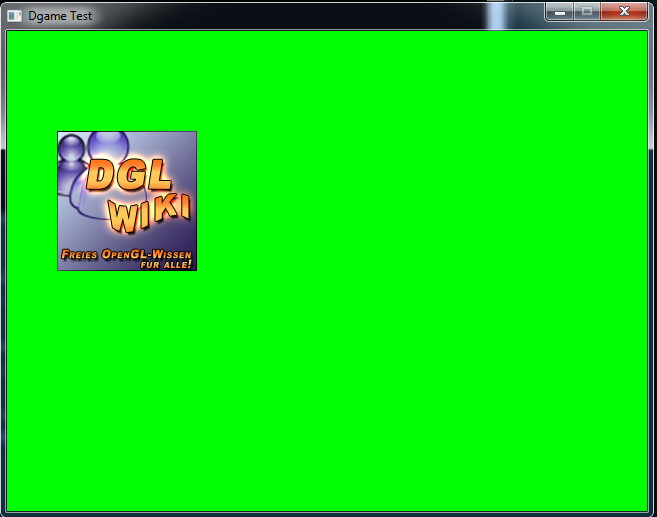Now the fun begins! We load images and put them into Sprites.

To load images, Dgame offers the Surface struct and Texture struct. For Sprites you have the Sprite class and the Spritesheet class.

In the following code we will load an image. Therefore we will use a Surface. The Surface is then given to a Texture (which loads the memory into an OpenGL Texture) which is then given to a Sprite, which also takes a position. After that the Sprite will be drawn onto the Window. Let's start.

Show Raw
```import std.stdio;

import Dgame.Window.Window;
import Dgame.Window.Event;
import Dgame.Graphic;

void main() {
Window wnd = Window(640, 480, "Dgame Test");
wnd.setClearColor(Color4b.Green);

Surface wiki_img = Surface("samples/images/wiki.png");
wiki_img.saveToFile("samples/images/wiki_clone.png");
Texture wiki_tex = Texture(wiki_img);

Sprite wiki = new Sprite(wiki_tex);
wiki.setPosition(50, 100);

bool running = true;

Event event;
while (running) {
wnd.clear();

while (wnd.poll(&event)) {
switch (event.type) {
case Event.Type.Quit:
writeln("Quit Event");

running = false;
break;

default: break;
}
}

wnd.draw(wiki);

wnd.display();
}
}```To do that, we call the Surface constructor with the filename. This loads the image and stores it. After that we make sure that it has really been loaded. Therefore we save it under a `*_clone` filename.
At this point we still cannot draw the Surface, because Surfaces have no idea where they should be drawn. This is the job of a Sprite. That's why we create a Sprite, which gets a Texture of the Surface. And now we can declare where the Sprite should be drawn. In this example on the coordinates 50x and 100y.
But: How do we draw it? That's pretty easy: we call `wnd.draw(wiki)`. That's the whole magic.

You should see something like this:But we can do more. We can move our Sprite, can declare which part of it should be drawn, check collisions with other Sprites and much more. Try it out.

To give you a pretaste, here is a snippet of how your Sprite bounces around in the Window.

Show Raw
```import std.stdio;

import Dgame.Window.Window;
import Dgame.Window.Event;
import Dgame.Graphic;
import Dgame.Math.Vector2;
import Dgame.Math.Rect : Size;

void main() {
Window wnd = Window(640, 480, "Dgame Test");
wnd.setVerticalSync(Window.VerticalSync.Enable);
wnd.setClearColor(Color4b.Green);

Texture wiki_tex = Texture(Surface("samples/images/wiki.png"));
Sprite wiki = new Sprite(wiki_tex);

int move_x = 4;
int move_y = 4;

bool running = true;

Event event;
while (running) {
wnd.clear();

while (wnd.poll(&event)) {
switch (event.type) {
case Event.Type.Quit:
writeln("Quit Event");

running = false;
break;

default: break;
}
}

const Vector2f pos = wiki.getPosition();
const Size wnd_size = wnd.getSize();

if (pos.x + wiki_tex.width >= wnd_size.width || pos.x < 0)
move_x *= -1;
if (pos.y + wiki_tex.height >= wnd_size.height || pos.y < 0)
move_y *= -1;

wiki.move(move_x, move_y);

wnd.draw(wiki);
wnd.display();
}
}```